# Frank solutions for Class 9 Maths ICSE chapter 11 - Triangles and their congruency [Latest edition]

#### Chapters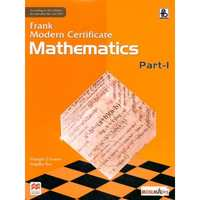## Chapter 11: Triangles and their congruency

Exercise 11.1Exercise 11.2
Exercise 11.1

### Frank solutions for Class 9 Maths ICSE Chapter 11 Triangles and their congruency Exercise 11.1

Exercise 11.1 | Q 1

In the given figure, ∠Q: ∠R = 1: 2. Find:
a. ∠Q
b. ∠R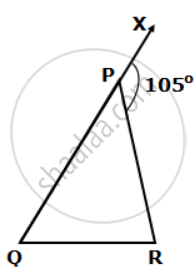Exercise 11.1 | Q 2

The exterior angles, obtained on producing the side of a triangle both ways, are 100° and 120°. Find all the angles of the triangle.

Exercise 11.1 | Q 3

Use the given figure to find the value of x in terms of y. Calculate x, if y = 15°.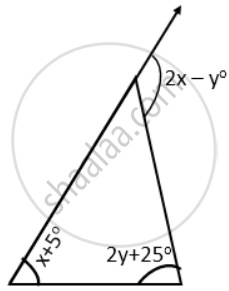Exercise 11.1 | Q 4

In a triangle PQR, ∠P + ∠Q = 130° and ∠P + ∠R = 120°. Calculate each angle of the triangle.

Exercise 11.1 | Q 5

The angles of a triangle are (x + 10)°, (x + 30)° and (x - 10)°. Find the value of 'x'. Also, find the measure of each angle of the triangle.

Exercise 11.1 | Q 6

Use the given figure to find the value of y in terms of p, q and r.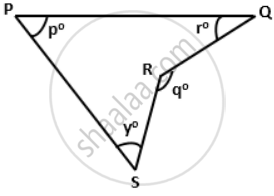Exercise 11.1 | Q 7

In the figure given below, if RS is parallel to PQ, then find the value of ∠y.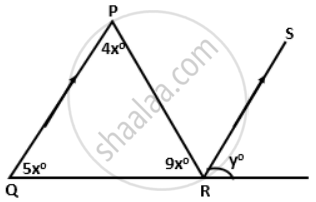Exercise 11.1 | Q 8

In a triangle PQR, the internal bisectors of angles Q and R meet at A and the external bisectors of the angles Q and R meet at B. Prove that: ∠QAR + ∠QBR = 180°.

Exercise 11.1 | Q 9

Use the given figure to show that: ∠p + ∠q + ∠r = 360°.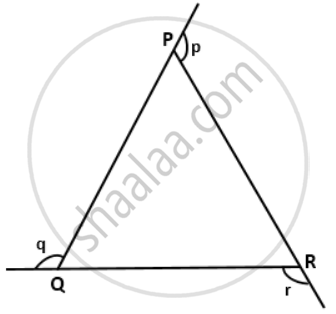Exercise 11.1 | Q 10

In a triangle ABC. If D is a point on BC such that ∠CAD = ∠B, then prove that: ∠ADC = ∠BAC.

Exercise 11.1 | Q 11

In a triangle ABC, if the bisectors of angles ABC and ACB meet at M then prove that: ∠BMC = 90° + (1)/(2) ∠A.

Exercise 11.1 | Q 12

If bisectors of angles A and D of a quadrilateral ABCD meet at 0, then show that ∠B + ∠C = 2 ∠AOD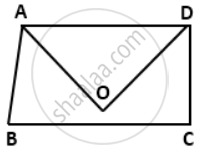Exercise 11.1 | Q 13

If each angle of a triangle is less than the sum of the other two angles of it; prove that the triangle is acute-angled.

Exercise 11.1 | Q 14

If the angles of a triangle are in the ratio 2: 4: 6; show that the triangle is a right-angled triangle.

Exercise 11.1 | Q 15

In a triangle, the sum of two angles is 139° and their difference is 5°; find each angle of the triangle.

Exercise 11.1 | Q 16

In a right-angled triangle ABC, ∠B = 90°. If BA and BC produced to the points P and Q respectively, find the value of ∠PAC + ∠QCA.

Exercise 11.2

### Frank solutions for Class 9 Maths ICSE Chapter 11 Triangles and their congruency Exercise 11.2

Exercise 11.2 | Q 1.1

Which of the following pairs of triangles are congruent? Give reasons
ΔABC;(BC = 5cm,AC = 6cm,∠C = 80°);
ΔXYZ;(XZ = 6cm,XY = 5cm,∠X = 70°).

Exercise 11.2 | Q 1.2

Which of the following pairs of triangles are congruent? Give reasons
ΔABC;(AB = 8cm,BC = 6cm,∠B = 100°);
ΔPQR;(PQ = 8cm,RP = 5cm,∠Q = 100°).

Exercise 11.2 | Q 1.3

Which of the following pairs of triangles are congruent? Give reasons
ΔABC;(AB = 5cm,BC = 7cm,CA = 9cm);
ΔKLM;(KL = 7cm,LM = 5cm,KM = 9cm).

Exercise 11.2 | Q 1.4

Which of the following pairs of triangles are congruent? Give reasons
ΔABC;(∠B = 70°,BC = 6cm,∠C = 50°);
ΔXYZ;(∠Z = 60°,XY = 6cm,∠X = 70°).

Exercise 11.2 | Q 1.5

Which of the following pairs of triangles are congruent? Give reasons
ΔABC;(∠B = 90°,BC = 6cm,AB = 8cm);
ΔPQR;(∠Q = 90°,PQ = 6cm,PR = 10cm).

Exercise 11.2 | Q 2

A is any point in the angle PQR such that the perpendiculars drawn from A on PQ and QR are equal. Prove that ∠AQP = ∠AQR.

Exercise 11.2 | Q 3

In the given figure P is a midpoint of chord AB of the circle O. prove that OP ^ AB.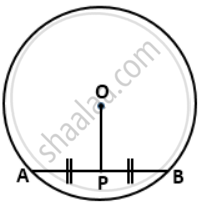Exercise 11.2 | Q 4

In a circle with center O. If OM is perpendicular to PQ, prove that PM = QM.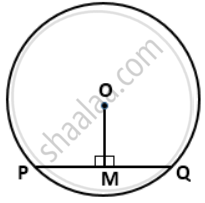Exercise 11.2 | Q 5

In ΔABC and ΔPQR and, AB = PQ, BC = QR and CB and RQ are extended to X and Y respectively and ∠ABX = ∠PQY. = Prove that ΔABC ≅ ΔPQR.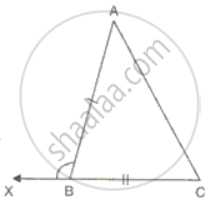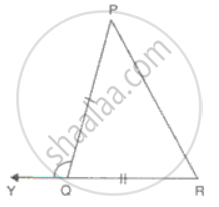Exercise 11.2 | Q 6

In a triangle ABC, if D is midpoint of BC; AD is produced upto E such as DE = AD, then prove that:
a. DABD andDECD are congruent.
b. AB = EC
c. AB is parallel to EC

Exercise 11.2 | Q 7

In the figure, ∠CPD = ∠BPD and AD is the bisector of ∠BAC. Prove that ΔCAP ≅ ΔBAP and CP = BP.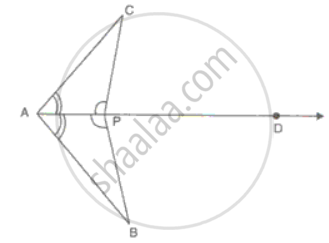Exercise 11.2 | Q 8

In the figure, BC = CE and ∠1 = ∠2. Prove that ΔGCB ≅ ΔDCE.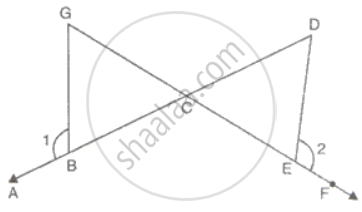Exercise 11.2 | Q 9

In ΔABC, AB = AC and the bisectors of angles B and C intersect at point O.Prove that BO = CO and the ray AO is the bisector of angle BAC.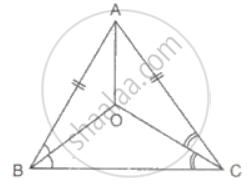Exercise 11.2 | Q 10

In the figure, AB = EF, BC = DE, AB and FE are perpendiculars on BE. Prove that ΔABD ≅ ΔFEC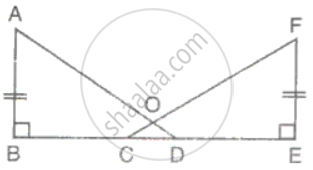Exercise 11.2 | Q 11

In the figure, BM and DN are both perpendiculars on AC and BM = DN. Prove that AC bisects BD.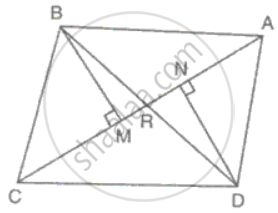Exercise 11.2 | Q 12

In ΔPQR, LM = MN, QM = MR and ML and MN are perpendiculars on PQ and PR respectively. Prove that PQ = PR.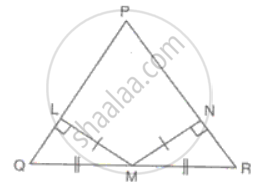Exercise 11.2 | Q 13

In the figure, RT = TS, ∠1 = 2∠2 and ∠4 = 2∠3. Prove that ΔRBT ≅ ΔSAT.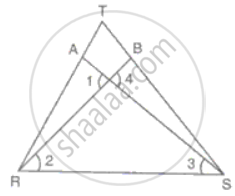Exercise 11.2 | Q 14

AD and BE are altitudes of an isosceles triangle ABC with AC = BC. Prove that AE = BD.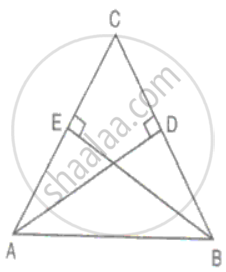Exercise 11.2 | Q 15

In ΔABC, X and Y are two points on AB and AC such that AX = AY. If AB = AC, prove that CX = BY.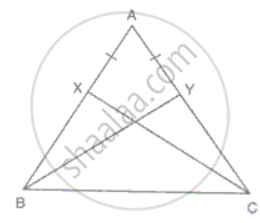Exercise 11.2 | Q 16

If the perpendicular bisector of the sides of a triangle PQR meet at I, then prove that the line joining from P, Q, R to I are equal.

Exercise 11.2 | Q 17

In the figure, AC = AE, AB = AD and ∠BAD = ∠EAC. Prove that BC = DE.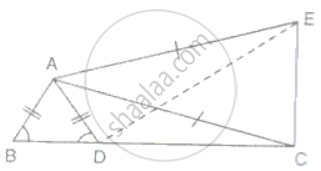Exercise 11.2 | Q 18

In the given figure ABCD is a parallelogram, AB is Produced to L and E is a midpoint of BC. Show that: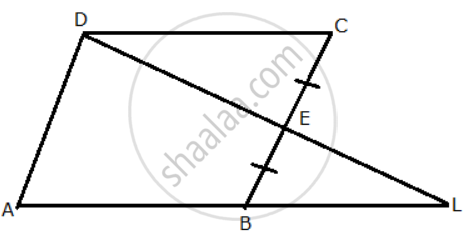a. DDCE ≅ DLDE
b. AB = BL
c. DC = "AL"/(2)

Exercise 11.2 | Q 19

In the figure, ∠BCD = ∠ADC and ∠ACB =∠BDA. Prove that AD = BC and ∠A = ∠B.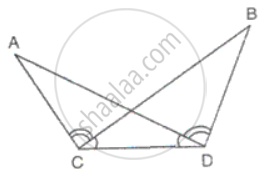Exercise 11.2 | Q 20

In the figure, AP and BQ are perpendiculars to the line segment AB and AP = BQ. Prove that O is the mid-point of the line segments AB and PQ.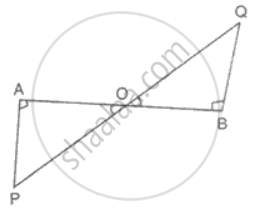Exercise 11.2 | Q 21

ΔABC is isosceles with AB = AC. BD and CE are two medians of the triangle. Prove that BD = CE.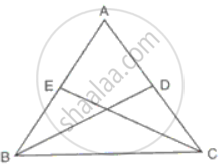Exercise 11.2 | Q 23

Sides, AB, BC and the median AD of ΔABC are equal to the two sides PQ, QR and the median PM of ΔPQR. Prove that ΔABC ≅ ΔPQR.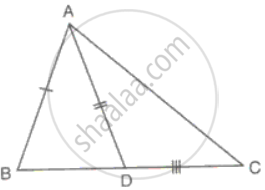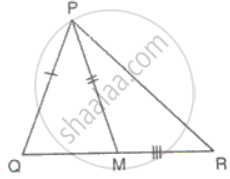Exercise 11.2 | Q 24

Prove that in an isosceles triangle the altitude from the vertex will bisect the base.

Exercise 11.2 | Q 25

In ΔABC, AB = AC. D is a point in the interior of the triangle such that ∠DBC = ∠DCB. Prove that AD bisects ∠BAC of ΔABC.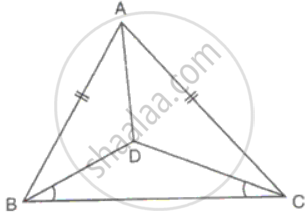Exercise 11.2 | Q 26

O is any point in the ΔABC such that the perpendicular drawn from O on AB and AC are equal. Prove that OA is the bisector of ∠BAC.

Exercise 11.2 | Q 27

In ΔABC, AB = AC, BM and Cn are perpendiculars on AC and AB respectively. Prove that BM = CN.

Exercise 11.2 | Q 28

ΔABC is an isosceles triangle with AB = AC. GB and HC ARE perpendiculars drawn on BC.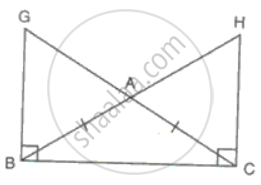Prove that
(i) BG = CH
(ii) AG = AH

Exercise 11.2 | Q 29

In ΔABC, AD is a median. The perpendiculars from B and C meet the line AD produced at X and Y. Prove that BX = CY.

Exercise 11.2 | Q 30

Two right-angled triangles ABC and ADC have the same base AC. If BC = DC, prove that AC bisects ∠BCD.

Exercise 11.2 | Q 31

PQRS is a quadrilateral and T and U are points on PS and RS respectively such that PQ = RQ, ∠PQT = ∠RQU and ∠TQS = ∠UQS. Prove that QT = QU.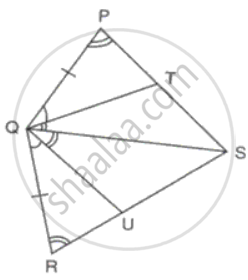Exercise 11.2 | Q 32

In the given figure, AB = DB and AC = DC. Find the values of x and y.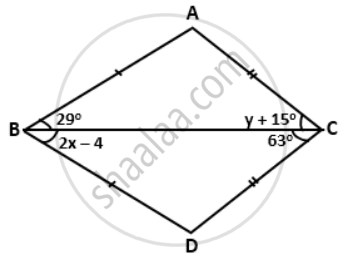## Chapter 11: Triangles and their congruency

Exercise 11.1Exercise 11.2## Frank solutions for Class 9 Maths ICSE chapter 11 - Triangles and their congruency

Frank solutions for Class 9 Maths ICSE chapter 11 (Triangles and their congruency) include all questions with solution and detail explanation. This will clear students doubts about any question and improve application skills while preparing for board exams. The detailed, step-by-step solutions will help you understand the concepts better and clear your confusions, if any. Shaalaa.com has the CISCE Class 9 Maths ICSE solutions in a manner that help students grasp basic concepts better and faster.

Further, we at Shaalaa.com provide such solutions so that students can prepare for written exams. Frank textbook solutions can be a core help for self-study and acts as a perfect self-help guidance for students.

Concepts covered in Class 9 Maths ICSE chapter 11 Triangles and their congruency are Relation Between Sides and Angles of Triangle, Important Terms of Triangle, Congruence of Triangles, Criteria for Congruence of Triangles, Concept of Triangles - Sides, Angles, Vertices, Interior and Exterior of Triangle.

Using Frank Class 9 solutions Triangles and their congruency exercise by students are an easy way to prepare for the exams, as they involve solutions arranged chapter-wise also page wise. The questions involved in Frank Solutions are important questions that can be asked in the final exam. Maximum students of CISCE Class 9 prefer Frank Textbook Solutions to score more in exam.

Get the free view of chapter 11 Triangles and their congruency Class 9 extra questions for Class 9 Maths ICSE and can use Shaalaa.com to keep it handy for your exam preparation